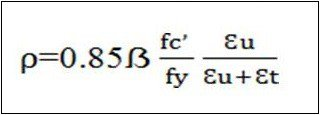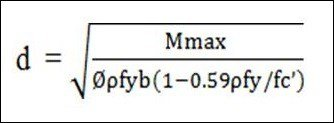# One Way Slab Design – How To Design One Way Slab

In this article, I will discuss how to design One way slab with example.

## What Is One Way Slab?

When a slab is supported on all four sides and the ratio of long span to short span is equal or greater than two, it will be considered as one way slab. The load on the slab is carried by the short span in one direction. However main reinforcement bar and distribution bar in transverse direction.

Longer span (l)/Shorter span (b) ≥ 2

## 1. Minimum Slab Thickness:

To control deflection, ACI Code 9.5.2.1 specifies minimum thickness values for one-way solid slabs.## 2. Span:

According to ACI code 8.7.1 If the slab rests freely on its supports, the span length may be taken as equal to the clear span plus the depth of the slab but need not exceed the distance between centers of supports.

## 3. Bar Spacing:

The lateral spacing of the flexural bars should not exceed 3 times the thickness h or 18 inch
according to ACI code 7.6.5 The lateral spacing of temperature and shrinkage reinforcement should not be placed farther apart than 5 times the slab thickness or 18 inch according to ACI code 7.12.2

READ -  Design Steps Of Simply Supported Steel Beam

## 4. Maximum Reinforcement Ratio:

Reinforcement ratio is the ratio of reinforcement area to gross concrete area based on total
depth of slab. One-way solid slabs are designed as rectangular sections subjected to shear and moment. Thus, the maximum reinforcement ratio corresponds to a net tensile strain in the
reinforcement, €t of 0.004

## 5. Minimum Reinforcement Ratio:

### a) For temperature and shrinkage reinforcement :

According to ACI Code 7.12.2.1

Slabs with Grade 40 or 50 deformed bars –> 0.0020

Slabs with Grade 60 deformed bars –> 0.0018

Slabs where reinforcement with yield strength Exceeding 60000 psi- ->( 0.0018 x 60000/fy)

### b) For flexural reinforcement :

According to ACI Code 10.5.4, the minimum flexural reinforcement is not to be less than the shrinkage reinforcement, or 0.0018

## Example Problem:

A reinforced concrete slab is built integrally with its supports and consists of equal span of 15 ft. The service live load is 100 psf and 4000 psi concrete is specified for use with steel with a yield stress equal to 60000 psi. Design the slab following the provisions of the ACI code.## Thickness Estimation:

For being both ends continuous minimum slab thickness =  L/28 =(15 x 120)/28= 6.43 in.
Let a trial thickness of 6.50 in.

Consider only a 1 ft width of beam.

Factored DL and LL ={81+1.2+(100 x 1.6)} =257 psf

## Determine Maximum Moments:

Factored moments at critical sections by ACI code :

At interior support : -M=1/9 x 0.257 x 152 = 6.43 k-ft

At midspan : +M=1/14 x 0.257 x 152 = 4.13 k-ft

At exterior support : -M=1/24 x 0.257 x 152 = 2.41 k-ft

Mmax = 6.43 k-ft=0.85 x 0.85 x 4/60 x 0.003/(0.003+0.004)

= 0.021

Now,= 2.64 in

## Check For Availability Of Thickness:

As ‘d’ is less than the effective depth of (6.50-1.00) = 5.50 in, the thickness of 6.50 in

## Reinforcement Calculation:

Let, a = 1 inch

At interior support:Checking the assumed depth of a bySimilarly at Midspan:

As = (4.13 x 12)/(0.90 x 60 x 5.29) = 0.17 in²

At Exterior support:

As = (2.41 x 12)/(0.90 x 60 x 5.29) = 0.10 in²

## Minimum Reinforcement:

As = 0.0018 x 12 x 6.50 = 0.14 in²

So we have to provide this amount of reinforcement where As is less than 0.14 in².

## Shrinkage Reinforcement:

Minimum reinforcement for shrinkage and temperature is

As = 0.0018 x 12 x 6.50 = 0.14 in²

## Final Design:## Layout Of One Way Slab: